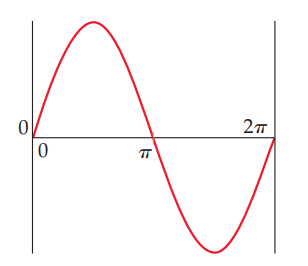×
Get Full Access to Chemistry: The Central Science - 14 Edition - Chapter 6 - Problem 6.8
Get Full Access to Chemistry: The Central Science - 14 Edition - Chapter 6 - Problem 6.8

×

# ?Consider a fictitious one-dimensional system with one electron. The wave function for the electron, drawn below, is $$\psi(x)=\sin x$$ from x = 0 to \ISBN: 9780134414232 1274

## Solution for problem 6.8 Chapter 6

Chemistry: The Central Science | 14th Edition

• Textbook Solutions
• 2901 Step-by-step solutions solved by professors and subject experts
• Get 24/7 help from StudySoup virtual teaching assistantsChemistry: The Central Science | 14th Edition

4 5 1 239 Reviews
16
2
Problem 6.8

Consider a fictitious one-dimensional system with one electron. The wave function for the electron, drawn below, is $$\psi(x)=\sin x$$ from x = 0 to $$x=2 \pi$$.

(a) Sketch the probability density, $$\psi^{2}(x)$$, from x = 0 to $$x=2 \pi$$.

(b) At what value or values of x will there be the greatest probability of finding the electron?

(c) What is the probability that the electron will be found at $$x=\pi$$? What is such a point in a wave function called? [Section 6.5]Text Transcription:

\psi(x)=\sin x

x=2 \pi

\psi^{2}(x)

x=\pi

Step-by-Step Solution:

Step 1 of 5) In some ways, the electrons in molecules behave like these balloons. We have seen that a single covalent bond is formed between two atoms when a pair of electrons occupies the space between the atoms. (Section 8.3) A bonding pair of electrons thus defines a region in which the electrons are most likely to be found. We will refer to such a region as an electron domain. Likewise, a nonbonding pair (or lone pair) of electrons, which was also discussed in Section 8.3, defines an electron domain that is located principally on one atom. For example, the Lewis structure of NH3 has four electron domains around the central nitrogen atom (three bonding pairs, represented as usual by short lines, and one nonbonding pair, represented by dots): Each multiple bond in a molecule also constitutes a single electron domain. Thus, the following resonance structure for O3 has three electron domains around the central oxygen atom (a single bond, a double bond, and a nonbonding pair of electrons):

Step 2 of 2

## Discover and learn what students are asking

Statistics: Informed Decisions Using Data : The Randomized Complete Block Design
?Given the following ANOVA output, answer the questions that follow: (a) The researcher wants to test H0: m1 = m2 = m3 =

Unlock Textbook Solution

?Consider a fictitious one-dimensional system with one electron. The wave function for the electron, drawn below, is $$\psi(x)=\sin x$$ from x = 0 to \